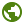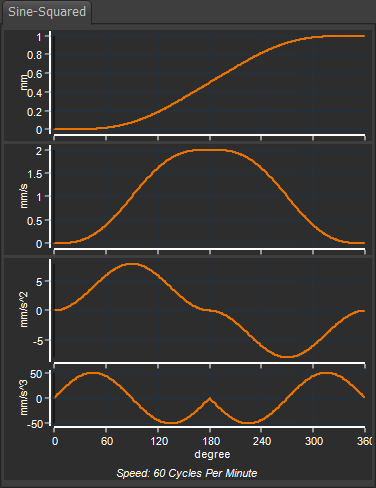﻿ MotionDesigner Reference & User Interface > Motion Laws / Cam-Laws for Segments > Sine-Squared Motion-Law

# Sine-Squared Motion-Law

### Sine-Squared Cam-Law, Motion-Law

Motion Description

 This maximum velocity and maximum acceleration are large when compared with other motion-laws. However, it has zero crossover jerk. At the start and end of this Motion-Law, the Y-axis does not change very much with a significant X–axis change. E.g. 0.02% Y-axis after 5% X-axis change. Therefore, to accurately reproduce the Motion-Law, it needs precise machining, a large cam, or an encoder with a high resolution. If possible, increase the by ~20% to reduce the maximum Velocity, Acceleration, and Jerk values.

#### Motion-Values

You CAN control the:

 Start Position The Start-Position usually flows from the End-Position of the End Position

You CANNOT control the:

 Start Velocity and End Velocity Start Acceleration and End Acceleration Start Jerk and End Jerk

Segment Parameters

 None

Segment-Range

 None

See also :See also :Tutorial 9: Asymmetrical Motions.Sine-Squared Motion-Law / Cam-Law

Motion-Law Coefficients

 Velocity Coefficient : Acceleration Coefficient : Jerk Coefficient : Jerk at Crossover :

Application Notes

 Dynamic Performance: This Motion-Law is recommended in applications where the period ratio is greater than 10. Pressure Angle Considerations This has a relatively large maximum-acceleration and maximum velocity, which increases the maximum pressure-angle. Drive Torques It has a high peak torque.